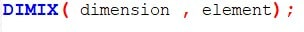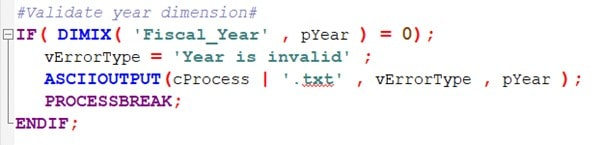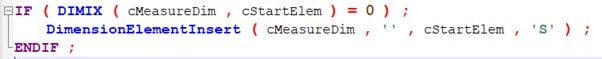# IBM Planning Analytics: DIMIX Function – When and how to use it

December 23, 2020

The DIMIX function is a helpful function that can be used in TurboIntegrator (TI) processes and TM1 rules. Simply put, the function will return the index number of an element within a dimension. If the element does not exist in the dimension, the function will return with a 0.The first argument in the function should reference the dimension you are working with, and the second argument is the element that is being checked.

In most contexts, this function will be used to be check whether an element exists in a dimension and the result can be used to set conditions for further logic.

This article will review a few of the most common ways DIMIX can be used, but there are many more uses cases beyond what is covered here.

Parameter ValidationThe code above is an example of a validation check against bad parameter inputs from within a TI process. pYear represents the parameter we are checking (e.g. 2009). DIMIX is being used to make sure the input (pYear) exists within the dimension called “Fiscal_Year”.   If the input parameter value does not exist within the dimension, the result will return “0” and the remaining code will create an error log and end the process.Another common use of DIMIX is to use a TI Process to add a missing element into a dimension if it does not already exist.  In the above code, the process first checks to see if the element, defined by the variable cStartElem, exists within the dimension, defined by the variable cMeasureDim.  If the result is “0” (meaning the element does NOT exist), the process will then insert the element into the dimension.  This proves useful in the Metadata tab of a TurboIntegrator process to avoid generating errors when loading data.